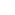Part of the Oxford Instruments Group
Expand

Shielding an X-ray Tube

Summary

One of the most important safety considerations (along with the high voltage) in operating your X-ray tube from Oxford Instruments is how much shielding you should use to contain radiation. Shielding an X-ray tube involves a simple calculation based on mass attenuation coefficients for different materials, described below.

Disclaimer

Oxford Instruments does not make any claim that these calculated values will result in adequate attenuation. Due to material and geometry differences, these values may only be used as a starting point for your application. You must test your setup with a reliable dosimeter to ensure safety.

X-Ray Mass Attenuation Theory

This application note assumes a beam of monochromatic photons with an incident intensity I0 that penetrates a material with mass thickness x and density. This beam will emerge with an intensity I given by the law1

I/ I0 = exp[-(μ/)x]

Values of μ/ have been empirically obtained using this equation and measured values of I0, I, and x. These values are compiled in the NIST X-Ray Mass Attenuation Coefficients2 and are used for all the calculations in this document. Note that mass thickness is defined as the mass per unit area, and is obtained by multiplying the thickness t by the density such that x = t.

We have completed the shielding calculations with a simple spreadsheet application for various materials using the theory above. The table below shows the shielding values for various materials. (Note that the Brass in this calculation is composed of 65% Copper, 33.5% Zinc, and 1.5% Lead). As a secondary consideration, these values have been crosschecked using existing Oxford Instruments XT experimental equipment.

For composite materials such as Brass, the mass attenuation coefficients are obtained using a weighted average:

μ/  =  wn(μ/)n

Here wn is the fraction by weight of the nth element in the material and, similarly, (μ/)n is the mass attenuation coefficient of the nth element in the material.

We have completed the shielding calculations with a simple spreadsheet application for various materials using the theory above. The table below shows the shielding values for various materials. (Note that the Brass in this calculation is composed of 65% Copper, 33.5% Zinc, and 1.5% Lead). As a secondary consideration, these values have been crosschecked using existing Oxford Instruments XT experimental equipment.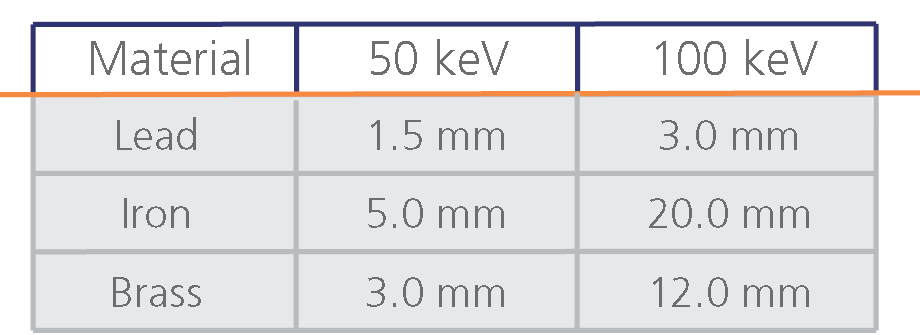The following charts show the transmission characteristics by varying material thickness.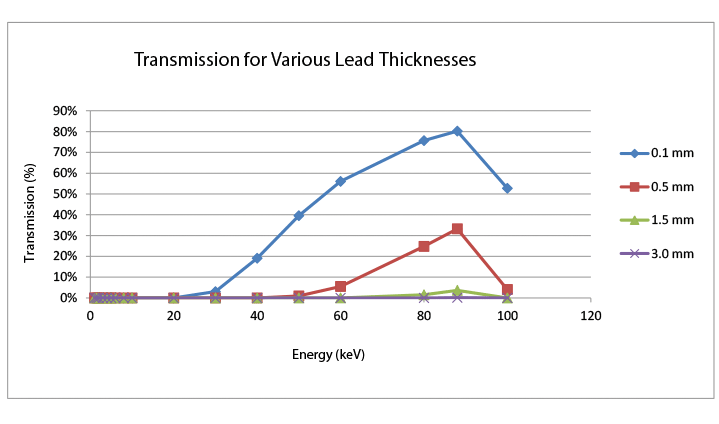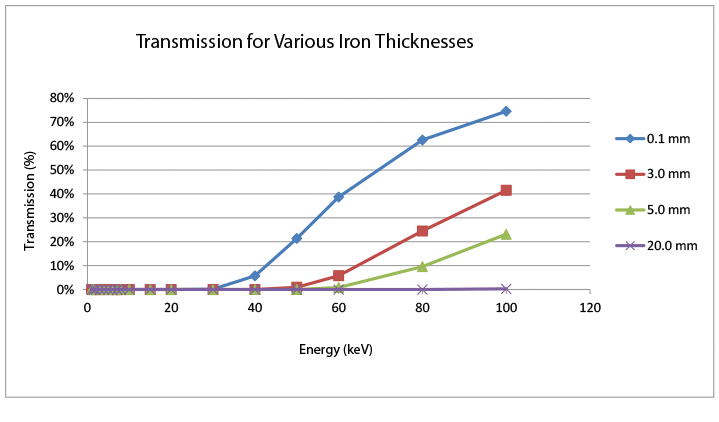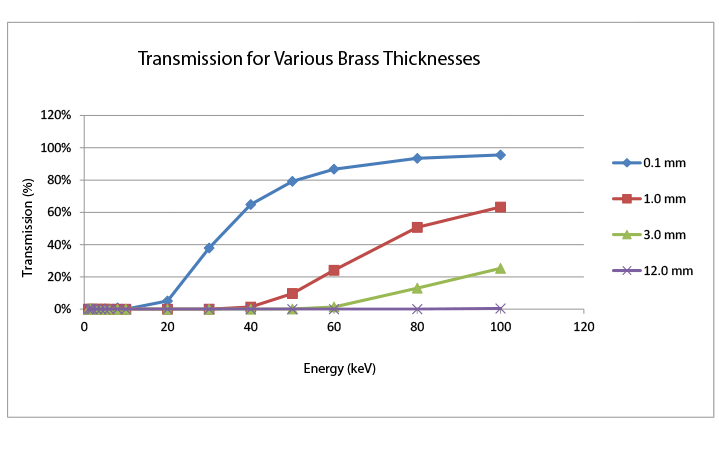Conclusion

To ensure safety, it is extremely important to adequately shield the outside environment from X­rays that are being emitted from your X­ray tube. In order to do this, we recommend starting with at least the thickness of materials shown above and measuring the output with a dosimeter. Again, while these values have been developed from first principles, it is imperative that you measure any setup thoroughly before putting it into full use.

References

1 http://www.physics.nist.gov/PhysRefData/XrayMassCoef/chap2.html

2 http://www.physics.nist.gov/PhysRefData/XrayMassCoef/tab3.html